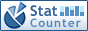Home PC Games Linux Windows Database Network Programming Server Mobile
 Home \ Database \ Oracle database NUMBER (x, y) data types - Using Vagrant to build multi-platform environment (Server) - Use Tmux and Vim to make IDE (Linux) - MySQL multi-instance configuration (Database) - Linux command find (Linux) - Linux excellent text editor (Markdown, LaTeX, MathJax) (Linux) - Hadoop 2.7.1 installation configuration based on availability QJM (Server) - Oracle 12C truncate table cascade (Database) - GROUP BY extension (Database) - Ubuntu install Geary (Linux) - MySQL 5.7 perfectly distributed transaction support (Database) - CentOS yum configuration under local sources (Linux) - xCAT deployment (Linux) - Linux SSH remote connection service slow Solutions (Linux) - GNU Linux system variables (sysctl configuration commands) integrated use (Linux) - Ubuntu install Lighttpd + Mono support .net (Linux) - Linux system security configuration (Linux) - To install Emacs under CentOS 6.5 (Linux) - Apache Web Security Linux systems (Linux) - Oracle Database High-Risk Vulnerability Warning (Database) - Mounting kit under Fedora Linux (Linux) Oracle database NUMBER (x, y) data types Add Date : 2017-08-31 Test preparation Analysis and experiment 1 fractional part of the processing 2 The maximum value that can be saved 3 The minimum value that can be saved to sum up Oracle's NUMBER data type represents a decimal number that is limited by the number of integer bits and the number of decimal places. Let's take NUMBER (8,2) as an example. 1 Test preparation To create a table with only one column, NUMBER (8,2) means that the total number of digits is at most 8 and the fractional part is at most 2 bits (the integer part is at most 8-2 = 6 bits). Create table t1 (c1 number (8,2)); 2 analysis and experiment 2.1 fractional part of the treatment The fractional part represents the degree of precision, NUMBER (8,2) can hold up to 2 decimal places, so what happens to more than 2 digits? SQL> insert into t1 values (1.234); 1 row created. SQL> select * from t1;         C1 ----------       1.23 SQL> delete from t1; 1 rows deleted. SQL> insert into t1 values (1.235); 1 row created. SQL> select * from t1;         C1 ----------       1.24 Can be seen that the fractional part of the decimal point after the 2-bit part of the rounding algorithm used, 1.234 1.23 as 1.23 and 1.24. 2.2 The maximum value that can be saved Since the integer part of up to 6, the fractional part of up to 2, then the number (8,2) can represent the largest number is 999999.99. We now insert this maximum value. SQL> insert into t1 values (999999.99); 1 row created. SQL> select * from t1;         C1 ----------  999999.99 This figure can be seen to be correctly stored. Insert a more large number 999999.991 below. SQL> insert into t1 values (999999.991); 1 row created. SQL> select * from t1;         C1 ----------  999999.99 Can be inserted, but was truncated to 999999.99, in fact, the reason is very simple fractional part of the 0.991 will be rounded to 0.99, after rounding the value became 999999.99, within the scope. Look at 999999.995. SQL> insert into t1 values (999999.995); Insert into t1 values (999999.995)                       * ERROR at line 1: ORA-01438: value greater than specified precision allowed for this column Insert failed! The reason is that the decimal part of 999999.995 becomes rounded to 1000000.00, beyond the 6-bit integer range. 2.3 The minimum value that can be saved The range of negative numbers is symmetric with the positive number range, so the minimum value is -999999.99. 3 Summary NUMBER (8,2) represents a range of numbers [-999999.99,999999.99]. To determine whether a given number can be in this range, the fractional part of the first rounded, and then to compare. Judgment step: (1) Round the third decimal place to obtain a number with 2 decimal places; (2) Determine whether the number is within the range of [-999999.99,999999.99]. More:
 CopyRight 2002-2022 newfreesoft.com, All Rights Reserved.